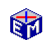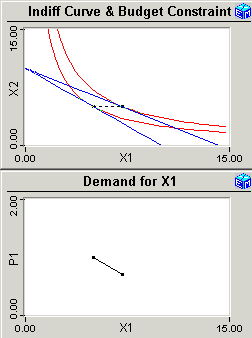Two Goods - Two Prices

The demand curve in the supply and demand model can be derived from the behavior of a utility maximizing consumer facing a budget constraint.  The agent's optimal consumption of a good depends on his income and on the price of the good.Model LinkTwo Goods - Two Prices
Printable PDF Exercises

The Two Goods - Two Prices application begins by challenging you to manually maximize utility given a budget constraint.  Tinkering with your consumption choices gives you an intuitive feel for the problem.  EconModel then shows you how to maximize utility with an automatic iterative procedure.These techniques are then used to derive the demand for the good X1.  You maximize utility given an initial price P1 for X1 and then change that price.  Graphing the resulting change in the optimal consumption of X1 traces out the demand for X1 as a function of its price P1.

Movie:  Derive a Demand Curve (32 seconds)

A follow-on exercise then decomposes the changes in the demand for X1 into income and substitution effects.

This analysis is then repeated for a complicated "translog" utility function, demonstrating that an optimizing agent (or an EconModel user!) can solve complicated problems without understanding the details of the math.

Classic Economic Models

Microeconomics

Introduction
Overview of Micro Models

Macroeconomics

Introduction
Overview of Macro Models

Resources